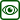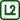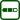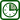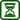## Refraction of Monochromatic Light With a Prism

### Experiment number : 1952

• #### Goal of experiment

This experiment should introduce to the students the term deviation angle and show them when the deviation angle is minimal.

• #### Theory

On the border of two media light reflects but it also refracts and passes from one medium to the other. Refraction is described by Snell’s law

n1sinα = n2sinβ,

where α os the angle of incidence, β the angle of refraction;n1, n2 are the corresponding refractive indices of the two media.

Optical prism deviates rays of light from their course by an angle δ, which we call the deviation angle. If a ray enters the prism from air with a refractive index n1 = 1, the direction of the ray after it passes through the prism will depend only on its apex angle φ and the refractive index n of the glass from which the prism is made. Passage of a ray through an optical prism is depicted in the picture below.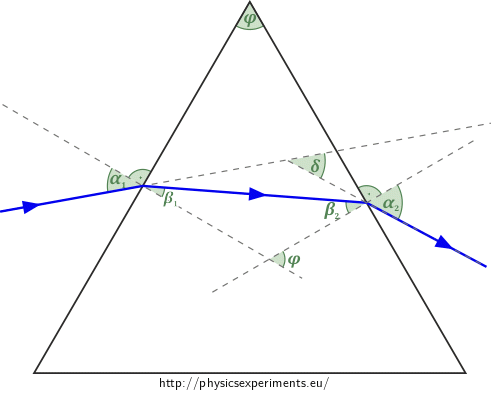We can express the deviation angle with relation

δ = (α1 - β1) + (α2 - β2) = α1 + α2 - φ.

Minimal deviation occurs when the trajectory of the ray is symmetrical, that is when α1 = α2 = α and β1 = β2 = β. For angle alfa for minimal deviation of a ray coming from air to the prism it holds that $\sin\alpha = n\sin\frac{φ}{2}.$

• #### Tools

Laser pointer, optical prism, paper, pencil, protractor.

• #### Procedure

1. We put the prism to the centre of our paper and mark the edges with the pencil.
2. We shine laser to the prism and mark the directions of incoming and outgoing rays with the pencil.
3. We prolong both rays and measure the angle between them.
4. We can repeat this procedure for different angles of incidence with a step of 10° and write down the values of each deviation angle.
5. From the measured values we can estimate at what angle of incidence is the deviation angle minimal.
6. If we know the refractive index of the material from which the prism is made and its apex angle, we can use the relation above to check the precision of our estimate.
• #### Technical notes

• Safety warning: When working with a laser pointer, it is important to follow safety rules. Light also reflects from the prism during the experiment, so we should observe it from the top to prevent eye damage.
• The experiment is very dependent on precision. Even small deviations while drawing the lines can have great effect on the result. Such imperfection is seen in the photo below.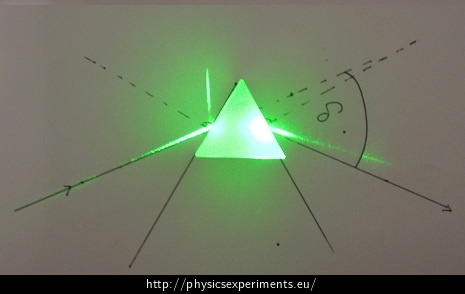• #### Pedagogical notes

• We can explain to students that light on the border partially reflects and partially refracts, as can be seen in the photo above.
• We can also use the prism to show the law of reflection.
• We can follow up the experiment with an explanation of how prisms are used to decompose white light into the colour spectrum.